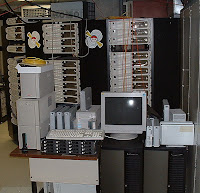### FlopsIn a recent astronomy talk, the speaker was discussing some sort of heavy-duty calculations, and how to make them faster. The way he put it, ultimately, was "flops are free". That is, CPUs are so fast now that they spend almost all their time waiting for data to be fetched from main memory: this means that the time it takes for a job to run is determined not by how many floating-point calculations it has to run but by how much data it must read from main memory.

This is kind of an astonishing statement, really: it says you can add floating-point calculations to your code at no extra cost. Of course such a statement can only be true for certain computing tasks on certain platforms, so I thought I'd test how true it is for a particular computing task I had in mind.

The task, you will probably not be surprised to discover, is pulsar searching. Specifically, I want to think about going through the Fourier transform of an observation looking for periodic signals.

Traditionally the way to do this is to take a giant FFT, square it to extract the power, and look for statistically-significant peaks. In fact, since pulsar signals are typically not just sine waves, one normally adds up the power from several harmonics and looks for statistically-significant peaks in the total power. There are lots of important details, but let's leave those aside for the moment. The idea I've been thinking about for a while now is based on a distinction.

When you estimate the total power in the harmonics, by a beautiful theorem in harmonic analysis, you are effectively estimating the root-mean-squared amplitude of the pulsar's folded pulse profile. But if you look at a profile that has one narrow peak, as many pulsars do, the peak-minus-mean amplitude can be much much more significant than the root-mean-squared amplitude. Back in the Fourier domain, when you have a single peak, not only is there power in many harmonics, but those harmonics line up in phase. The usual approach ignores the phases of the harmonics entirely. How much could be gained by paying attention to them?

Some informal experiments suggest that for a pulsar that is on only 1% of the time (which is not too rare among slow pulsars), this approach may offer something like a 40% improvement in sensitivity. Since that's the same as doubling the observation time, I think it's worth looking into.

As with everything, this improved sensitivity comes at a cost. In particular, all the codes we have to compute it are substantially slower than the code we have that uses the incoherent approach. So, thinking about how to speed things up, it occurred to me to look into just why the code was slow.

I'm sure there are brilliant and subtle tools to count cache misses and do nanosecond timing on running code, but I thought I'd take a more direct approach: just write dummy code that does only one thing and time it. In particular, what I want to compare is the time to extract 64 harmonics from a giant FFT to the time it takes to take an inverse FFT of those harmonics to reconstruct the profile.

I should say that my initial feeling was that the inverse FFT would dominate the time - after all, even an n log n algorithm requires a fair number of floating-point operations to produce a 256-point real inverse FFT. But the output is:

Array reading 1.71528 GB/sPlanning FFTRunning FFTsFFT time 1.57851e-06 secondsFFT rate 633510 /sFFT rate/read rate: 0.176112

That is, yes, the FFTs are the slow step, but only by a factor of five. That is, reading all those coefficients in from memory takes a fifth as long as doing all those FFTs. (Incidentally, if these numbers seem low, the machine I'm running this on is a couple of years old. Edit: it's not the machine pictured above, which is even older, and which does most of my number-crunching.) Here's the code:

#include <stdio.h>#include <stdlib.h>#include <time.h>#include <complex.h>#include <fftw3.h>const int repeats=20;const int array_size=1<<26;const int irfft_size=256;const int fft_batch=1<<18;int main(int argc, char*argv[]) {  double t, tnew;  float*array;  double sum;  double array_rate;  int i,j,k;  struct timeval start, end;  fftwf_plan plan;  fftwf_complex *in;  float *out;  array = (float*)malloc(array_size*sizeof(float));  if (!array) {      perror("Allocation failed");      return 1;  }  sum = 0;  t = 1e100;  for (j=0;j<repeats;j++) {      gettimeofday(&start,0);      for (i=0;i<array_size;i++)          sum += array[i];      gettimeofday(&end,0);      tnew = end.tv_sec-start.tv_sec+(end.tv_usec-start.tv_usec)/1e6;      if (tnew<t) t=tnew;  }  printf("Array reading time %g seconds\n", t, sum);  array_rate = array_size*sizeof(float)/t;  printf("Array reading %g GB/s\n", array_rate/(1<<30));  free(array);  in = (fftwf_complex*) fftwf_malloc(sizeof(fftwf_complex)*(irfft_size/2+1));  out = (float*) fftwf_malloc(sizeof(float)*irfft_size);  printf("Planning FFT\n");  plan = fftwf_plan_dft_c2r_1d(irfft_size, in, out, FFTW_MEASURE | FFTW_EXHAUSTIVE | FFTW_DESTROY_INPUT);  printf("Running FFTs\n");  t = 1e100;  for (j=0;j<repeats;j++) {      gettimeofday(&start,0);      for (i=0;i<fft_batch;i++) {          for (k=0;k<(irfft_size/2+1);k++) {              in[k] = 0;          }          fftwf_execute(plan);      }      gettimeofday(&end,0);      tnew = end.tv_sec-start.tv_sec+(end.tv_usec-start.tv_usec)/1e6;      if (tnew<t) t=tnew;  }  printf("FFT time %g seconds\n", t/fft_batch);  printf("FFT rate %g /s\n", fft_batch/t, sum);  printf("FFT rate/read rate: %g\n", (fft_batch/t)/(array_rate/(2*sizeof(float)*(irfft_size/4))));  fftwf_destroy_plan(plan);  fftwf_free(in);  fftwf_free(out);  return 0;}

Compiled with:
gcc -O9 -march=native -ffast-math -lfftw3f -lm timer.c

What this tells me is interesting. On the one hand, if I can do something really clever to make the FFTs faster, or avoid them, I can get some improvement, but sooner or later I'm going to hit the limit of memory loading. On the other hand, if I can't make them faster or fewer - and I probably can't - there's not much point sweating much over reducing the memory loads.

Anyway, the upshot of all this is: on modern hardware, you can do an awful lot of flops - a 256-point real FFT - for not much more than the cost of loading the data in from main memory. So if you have some clever mathematical trick to reduce your data size (interpolation, say) it may be worth implementing.